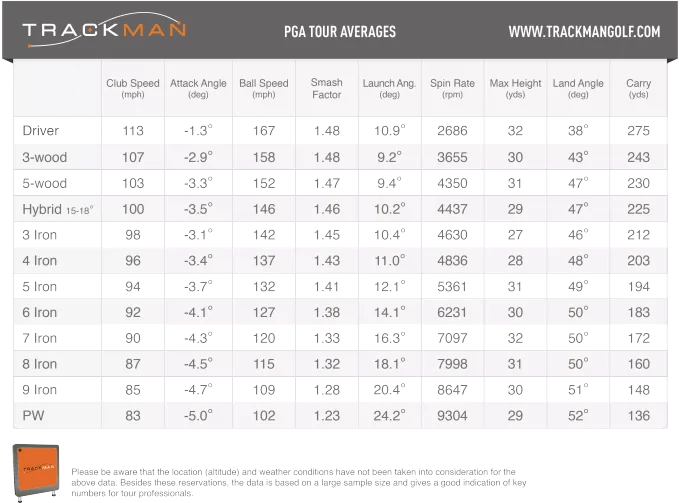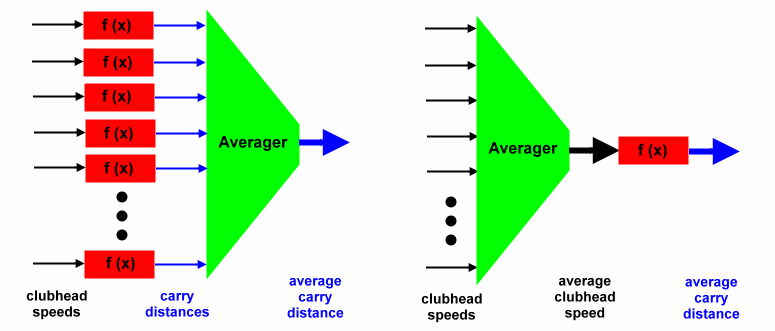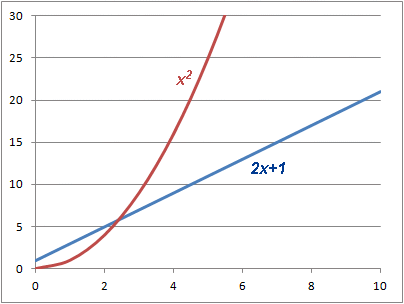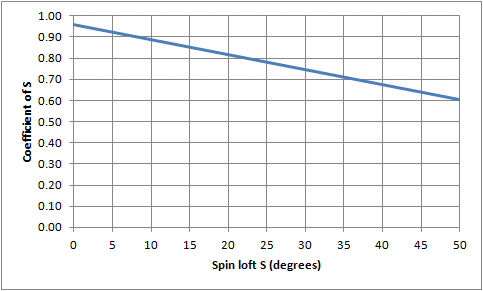# Does averaging stats work?

Dave Tutelman -- November 20, 2017

Does the average of the function equal the function of the average? That's an odd-sounding question, but it is important to know the answer for some kinds of golf research -- particularly when your data consists of averages and you want to use them to find or test a mathematical relationship.
Lots of times in golf research (or any kind of investigation), you want to test some mathematical function that lets you calculate how the world works. For instance, suppose we want to test the common assertion:

Carry distance = 2.5 * clubhead speedIt's worth noting this assertion is false, but you see it often enough in popular articles -- and for this discussion it has the advantage of simplicity. You want to test the function against real live data, which is exactly how you should test it! Unfortunately, unless you own a TrackMan or equivalent, it will be hard to get that data.

But wait! TrackMan publishes annually a table of data showing how tour players hit the ball. (The image is a snapshot directly from the TrackMan web page.) We should be able to use that data, right?

Not so fast! The formula works (or doesn't work) for a drive -- one drive. Measure the clubhead speed, measure the carry distance, and see if they are related by a factor of 2.5. But this chart isn't about individual shots. Each number there is an average of many shots by many different golfers. The shots had many different clubhead speeds and many different distances. Before we use the data from such combined-statistics charts, we need to answer the question: Does the average of the results come out to be the same number as the result of the average? If not then it is not valid to check the formula assuming each row is an individual golf shot.

The words may be confusing, so let's show the question visually. Let's consider a function to go from clubhead speed to carry distance. For instance, for the assertion above, the function would be f(x)=2.5x. Here are two ways we could use the function.In the diagram, clubhead speeds are black and carry distances are blue. Data for individual shots are thin arrows and averages are fat arrows.
• The calculation on the left takes each individual shot's clubhead speed and applies it to the function to get a carry distance. Then it averages the carry distance for a final answer. Mathematically, it is Avg(f(x)), the average of the function.
• The calculation on the right averages all the clubhead speeds, and applies the resulting average clubhead speed to the function to get a final answer. Mathematically, it is f(Avg(x)), the function of the average.
Do these two different calculations give the same answer? That is a very important question if we want to use charts of averages like the TrackMan chart or similar charts from the PGA Tour.
If they do give the same answer, then we can apply functions to the cells in the charts and expect them to make sense.
If they don't give the same answer, it isn't valid to use the data averages for anything interesting.

## Simple numerical examples

### Example #1: One that works

 x f(x)=2x+1 0 1 1 3 2 5 3 7 4 9 5 11 6 13 7 15 8 17 9 19 10 21 5 11
We'll compute the answer both ways. Excel makes the work trivially easy, so here is the spreadsheet direct from Excel. We have take individual data points -- values of x -- from zero to ten. (That is 0, 1, 2, 3,... 10) We apply the function f(x) to each data point individually; that is the second column. The blue cells at the bottom are the averages of the columns. Let's see how the calculations worked out.
• The average value of x is 5.
• The average value of f(x) is 11.
• If we apply the function f(x) to the average of x, we get the average of f(x). That is:
f(5) = 2*5 + 1 = 11
So for this function and this data distribution, it all works. I'll go further and say (without proving it here) that with this function it will work for any distribution of input data x.

### Example #2: One that doesn't work

 x f(x)=x2 0 0 1 1 2 4 3 9 4 16 5 25 6 36 7 49 8 64 9 81 10 100 5 35
So far the results are encouraging. Let's try another simple function: f(x)=x2
Again, we compute the answer both ways. This time:
• The average value of x is 5. (Not surprising; we used the same input distribution.)
• The average value of f(x) is 35.
• If we apply the function f(x) to the average of x, we get:
f(5) = 52 = 25
Whoops! We have a problem. 25 (the function of the average input) is not the same as 35 (the average of all the fuction outputs). What this means is that the function f(x)=x2 will not give valid results when applied to a table of data averages.

## GeneralizationWhy does it work this way? Can we tell anything about which functions will work and which will not?

Let's start by looking at a graph of the two functions we just tested. We see right away that:
• The one that worked (2x+1) is a straight line. The slope does not change from 2.0 over the range we tested.
• The one that didn't work (x2) has a lot of curvature. The slope is zero (horizontal) at x=0 and all the way up to 20 at x=10.
That is a critical difference. If the slope of the function does not change, the function is linear, and linear functions do not distort averaged data. If the function is curved -- if the slope changes -- then you can't trust it not to distort the data. It might give correct answers by coincidence, with the right data; but you can't depend on it, so it isn't valid to test mathematical relationships.

How can we tell whether the function we are using is linear? Remember when you learned to graph a straight line back in high school or perhaps even middle school? The form I learned for the equation at the time was:

y = mx + b

where m is the slope of the line and b is the place it crosses the y-axis. The function is linear if, the only places x appears in the function, it is only multiplied by a constant. No squares, no inverses, no exponents -- just multiplied by a constant. If we have a function of several variables (x1, x2,...), then the form for it to be linear is:

y = k0 + k1x1 + k2x2 + ...

where all the ks are constants. Functions in this form are linear, and will not distort data averages.

(For those who are interested, the proof is in the Appendix.)

## Realistic example

This topic came up most recently in a discussion of how to find launch angle from angle of attack (AoA) and dynamic loft. The golf community has gradually come to accept a relationship of the form:

L = A + p(D-A)

where L is launch angle, A is AoA, and D is dynamic loft. The percentage p is generally acknowledged to vary with the loft. (Specifically, it varies with spin loft, which is equal to D-A.) The person doing the work in this project had found a table of tour averages, and was trying to use it to validate the value of p for various clubs.

It is valid to use averages in this case?

Well, the function is certainly linear in D and A; each of those variables appear only multiplied by p or 1. But p varies with loft, so maybe it's a problem. Let's look at the variation of p.

I have curve-fit a formula for p as a function of spin loft S. Specifically, it is:

p  =  .96  -  .0071 S

If we apply that to the launch angle, and then S=D-A we get:

L  =  A + (.96 - .0071S)(D-A)
L  =  A + .96(D-A) - .0071(D-A)2

Hmmm! Looks like we have a square in the function. How badly will that mess up research based on averaged data?

Let's do some graphing. We'll set AoA to some harmless value (zero is pretty harmless). For zero AoA, the dynamic loft equals the spin loft. Here are graphs of the launch angle and its slope, plotted against spin loft. Remember that D is the same as S (since A=0), and the slope  of the curve is the coefficient p.It is clear from looking at launch angle that the function shows plenty of curvature. Remember, curvature is what distorts statistical distributions and invalidates using averages as if they were individual data points.

But there is a silver lining in the cloud of doubt here. The table of averaged data is grouped by club: driver, 3-wood, and down to pitching wedge. Each club has its own row of averaged data, and the averaging is confined to just one type of club. How does that help? If the averages are only over one type of club, that greatly limits the range of spin loft we have to consider.

For instance, the driver for a tour player is not likely to be outside the range of 10°-13° of spin loft. True, the clubhead's loft is lower than that for the static club, but shaft bend adds a little loft. In any event, it's about a 3° range. When we look at the slope on the graph on the right, a 3° range is about a 0.021 range of slope. For a driver, that's about a 2.4% change of slope over the range -- not much curvature at all. And you can tell that by eye as well, looking at the graph on the left. By eye, the curve from 10° to 13° is indistinguishable from a straight line.

So it's probably quite all right to use the data, as long as we confine our conclusion to one row of data at a time. Over the variation in that row, the function is linear, for practical purposes if not mathematically.

### Relationship to series expansions

If you took advanced algebra or calculus, here's an interesting way of looking at it. If you didn't, you won't miss much by not reading this part.

Do you remember expanding functions as Taylor's series? It looked like:

f(x)  =  k0  +  k1(x-a)  +  k2(x-a) +  k3(x-a)3  + ...

You could get a very good representation of any function in the vicinity of the point x=a. How good? For a given range around the point a, you can be arbitrarily good; you just have to take enough terms in the series. Example: for a range of 3≤x≤5 you want to use a=4; you might need to use a third-order series to represent your function accurately within 0.1%.

This is directly applicable to what we have here. For the range of interest, if we get a good representation of the function from a first-order Taylor series, then we can say the function is effectively linear over the range of interest. Why? Because a first-order series is a linear equation:

f(x)  =  k0  +  k1(x-a)

## Conclusions

In golf research (and other types of research as well) we often find data available in the form of averages over a lot of trials. It is seldom the raw data for each individual trial, unless we did the experiment ourselves. Can we use such averaged data to test or even derive mathematical relationships?
• If the mathematical relationship is linear in the data variables, then we can draw valid conclusions about individual trials from the averages.
• To the extent the mathematical relationship contains significant curvature, then any conclusions we draw about individual trials cannot be trusted.

## Proof that a linear function allows averaged inputs and outputs

The major idea in this article is that a linear function gives the same answer for the average of the function that it does for the function of the average, and that a nonlinear function cannot be trusted to give the same answer. The latter is easy to show; we already did when we looked at the second simple numerical example, f(x)=x2.  But we need to prove the former. So far we just showed that it works for one linear function with one distribution of data; that does not prove the general case. So here's a proof of the general case.

We'll start with the diagram we used at the beginning of the article, and the function we use will be the perfectly general linear function, y=mx+b. The data distribution will be a perfectly general set of xi. We work through the calculation for each side. When we have the result, we can see by inspection that they give the same answer.y  =  Avg ( f(xi) )

 y  = 1N NΣi=1 f(xi)

 y  = 1N NΣi=1 (mxi + b)

 y  = 1N NΣi=1 (mxi)  + 1N NΣi=1 b

 y  = mN NΣi=1 xi  + NN b

 y  = mN NΣi=1 xi  + b
y  =  f( Avg (xi) )

 y  =   f ( 1N NΣi=1 xi  )

 y  =   m( 1N NΣi=1 xi )   + b

 y  = mN NΣi=1 xi  + b

Both columns end with exactly the same expression, so both methods of calculation give the same result.